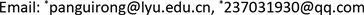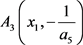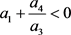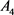﻿ 一类三次多项式系统的定性分析 Qualitative Analysis of a Class of Cubic Polynomial Systems

Dynamical Systems and Control
Vol. 08  No. 03 ( 2019 ), Article ID: 31552 , 8 pages
10.12677/DSC.2019.83022

Qualitative Analysis of a Class of Cubic Polynomial Systems

Wenjing Ding1*, Guirong Pan2*, Wenya Jiang1

1School of Mathematics and Statistics, Linyi University, Linyi Shandong

2School of Information Science and Engineering, Linyi University, Linyi ShandongReceived: Jul. 6th, 2019; accepted: Jul. 16th, 2019; published: Jul. 31st, 2019ABSTRACT

In this paper, the classical method of qualitative analysis is used to analyze the existence, type and local stability of a class of planar cubic polynomial differential system $\frac{dx}{dt}=-y+{a}_{1}x+{a}_{2}{x}^{2}+{a}_{3}{y}^{3}+{a}_{4}x{y}^{2}$ , $\frac{dy}{dt}=x\left(1+{a}_{5}y\right)$ . And the formal series method is used to determine the center-focus of the singular point. Finally, the conditions of the existence of limit cycles of the system are obtained by using the Hopf bifurcation method.

Keywords:Cubic Polynomial System, Singular Point, Limit Cycle, Hopf Bifurcation

1临沂大学数学与统计学院，山东 临沂

2临沂大学信息科学与工程学院，山东 临沂1. 引言(1)

2. 预备知识

2.1. 奇点定义  

2.2. 奇点的类型  

2.3. Hopf分支问题  

$\left\{\begin{array}{l}\frac{\text{d}x}{\text{d}t}=P\left(x,y,\lambda \right)\\ \frac{\text{d}y}{\text{d}t}=Q\left(x,y,\lambda \right)\end{array}$ (2)

3. 奇点分析

$\left\{\begin{array}{l}-y+{a}_{1}x+{a}_{2}{x}^{2}+{a}_{3}{y}^{3}+{a}_{4}x{y}^{2}=0\\ x\left(1+{a}_{5}y\right)=0\end{array}$ (3)

2) 若 ${a}_{3}>0$ ，则系统(1)有奇点 ${A}_{1}\left(0,{y}_{0}\right)$${A}_{2}\left(0,-{y}_{0}\right)$ ，其中 ${y}_{0}=\frac{1}{\sqrt{{a}_{3}}}$

3) 若 ${a}_{5}\ne 0$ ，则系统(1)有奇点${A}_{4}\left({x}_{2},-\frac{1}{{a}_{5}}\right)$ ，其中 ${x}_{1}=\frac{-\left({a}_{1}+\frac{{a}_{4}}{{a}_{5}^{2}}\right)+\sqrt{\Delta }}{2{a}_{2}}$${x}_{2}=\frac{-\left({a}_{1}+\frac{{a}_{4}}{{a}_{5}^{2}}\right)-\sqrt{\Delta }}{2{a}_{2}}$$\Delta ={\left({a}_{1}+\frac{{a}_{4}}{{a}_{5}^{2}}\right)}^{2}-4{a}_{2}\left(\frac{1}{{a}_{5}}-\frac{{a}_{3}}{{a}_{5}^{3}}\right)$

3.1. 奇点 $O\left(0,0\right)$

2) 当 ${a}_{1}\le -2$$O\left(0,0\right)$ 为系统(1)的稳定结点， ${a}_{1}\ge 2$ 时为不稳定结点。

3) 当 ${a}_{1}=0$$O\left(0,0\right)$ 为系统(1)的中心型奇点(中心或者细焦点)。

3.2. 奇点 ${A}_{1}\left(0,{y}_{0}\right)$

2) 若 ${a}_{5}>-\sqrt{{a}_{3}}$ ，则特征根为异号实根， ${A}_{1}\left(0,{y}_{0}\right)$ 为系统(1)的鞍点。

3) 若 ${a}_{5}<-\sqrt{{a}_{3}}$ ，则

i) 当 ${\left({a}_{1}+\frac{{a}_{4}}{{a}_{3}}\right)}^{2}+8\left(1+\frac{{a}_{5}}{\sqrt{{a}_{3}}}\right)>0$ 时特征根为同号实根， ${A}_{1}\left(0,{y}_{0}\right)$ 为系统(1)的结点， ${a}_{1}+\frac{{a}_{4}}{{a}_{3}}>0$ 时不稳定， ${a}_{1}+\frac{{a}_{4}}{{a}_{3}}<0$ 时稳定。

ii) 当 ${\left({a}_{1}+\frac{{a}_{4}}{{a}_{3}}\right)}^{2}+8\left(1+\frac{{a}_{5}}{\sqrt{{a}_{3}}}\right)<0$ 时特征根为共轭复根， ${A}_{1}\left(0,{y}_{0}\right)$ 为系统(1)的焦点， ${a}_{1}+\frac{{a}_{4}}{{a}_{3}}>0$ 时不稳定， ${a}_{1}+\frac{{a}_{4}}{{a}_{3}}<0$ 时稳定。 ${a}_{1}+\frac{{a}_{4}}{{a}_{3}}=0$${A}_{1}\left(0,{y}_{0}\right)$ 为系统(1)的中心型奇点(中心或者细焦点)。

3.3. 奇点 ${A}_{2}\left(0,-{y}_{0}\right)$

2) 若 ${a}_{5}<\sqrt{{a}_{3}}$ ，则特征根为异号实根， ${A}_{2}\left(0,-{y}_{0}\right)$ 为系统(1)的鞍点。

3) 若 ${a}_{5}>\sqrt{{a}_{3}}$ ，则

i) 当 ${\left({a}_{1}+\frac{{a}_{4}}{{a}_{3}}\right)}^{2}+8\left(1+\frac{{a}_{5}}{\sqrt{{a}_{3}}}\right)>0$ 时特征根为同号实根， ${A}_{2}\left(0,-{y}_{0}\right)$ 为系统(1)的结点， ${a}_{1}+\frac{{a}_{4}}{{a}_{3}}>0$ 时不稳定， ${a}_{1}+\frac{{a}_{4}}{{a}_{3}}<0$ 时稳定。

ii) 当 ${\left({a}_{1}+\frac{{a}_{4}}{{a}_{3}}\right)}^{2}+8\left(1+\frac{{a}_{5}}{\sqrt{{a}_{3}}}\right)<0$ 时特征根为共轭复根， ${A}_{2}\left(0,-{y}_{0}\right)$ 为系统(1)的焦点， ${a}_{1}+\frac{{a}_{4}}{{a}_{3}}>0$ 时不稳定，时稳定。 ${a}_{1}+\frac{{a}_{4}}{{a}_{3}}=0$${A}_{2}\left(0,-{y}_{0}\right)$ 为系统(1)的中心型奇点(中心或者细焦点)。

3.4. 奇点 ${A}_{3}\left({x}_{1},-\frac{1}{{a}_{5}}\right)$

2) 当 ${d}_{2}<0$ 时， ${A}_{3}$ 为系统的鞍点。

3) 若 ${d}_{2}>0$ ，则

i) 当 ${d}_{1}^{2}-4{d}_{2}\ge 0$ 时，特征根为同号实根， ${A}_{3}$ 为系统(1)的结点， ${d}_{1}>0$ 时不稳定， ${d}_{1}<0$ 时稳定。

ii) 若 ${d}_{1}^{2}-4{d}_{2}<0$ 时，特征根为共轭复根， ${A}_{3}$ 为系统(1)的焦点， ${d}_{1}>0$ 时不稳定， ${d}_{1}<0$ 时稳定。 ${d}_{1}=0$${A}_{3}$ 为系统(1)的中心型奇点(中心或者细焦点)。

3.5. 奇点 ${A}_{4}\left({x}_{2},-\frac{1}{{a}_{5}}\right)$

2) 当 ${d}_{4}<0$ 时， ${A}_{4}$ 为系统的鞍点。

3) 若 ${d}_{4}>0$ ，则

i) 当 ${d}_{3}^{2}-4{d}_{4}\ge 0$ 时，特征根为同号实根， ${A}_{4}$ 为系统(1)的结点， ${d}_{3}>0$ 时不稳定， ${d}_{3}<0$ 时稳定。

ii) 若 ${d}_{3}^{2}-4{d}_{4}<0$ 时，特征根为共轭复根，为系统(1)的焦点， ${d}_{3}>0$ 时不稳定，时稳定。 ${d}_{3}=0$${A}_{4}$ 为系统(1)的中心型奇点(中心或者细焦点)。

3.6. 细焦点

$\left\{\begin{array}{l}\frac{\text{d}y}{\text{d}t}=-y+{a}_{2}{x}^{2}+{a}_{3}{y}^{3}+{a}_{4}x{y}^{2}\\ \frac{\text{d}y}{\text{d}t}=x+{a}_{5}xy\end{array}$ (4)

2) 当 ${a}_{4}<0$ 时， $O\left(0,0\right)$ 是系统(4)的一阶稳定细焦点。

3) 当 ${a}_{4}=0$ 时， $O\left(0,0\right)$ 为中心。

$\begin{array}{c}{\frac{\text{d}F}{\text{d}t}|}_{\left(1\right)}=\frac{\partial F}{\partial x}\stackrel{˙}{x}+\frac{\partial F}{\partial y}\stackrel{˙}{y}\\ =\left(2x+\frac{\partial {F}_{3}}{\partial x}+\frac{\partial {F}_{4}}{\partial x}+\cdots \right)\left(-y+{a}_{2}{x}^{2}+{a}_{3}{y}^{3}+{a}_{4}x{y}^{2}\right)\\ \text{\hspace{0.17em}}\text{\hspace{0.17em}}+\left(2y+\frac{\partial {F}_{3}}{\partial y}+\frac{\partial {F}_{4}}{\partial y}+\cdots \right)\left(x+{a}_{5}xy\right)\end{array}$ (5)

$\frac{\text{d}{F}_{3}}{\text{d}\theta }=-2\left({a}_{2}{\mathrm{cos}}^{3}\theta +{a}_{5}\mathrm{cos}\theta {\mathrm{sin}}^{2}\theta \right)$ (6)

${\int }_{0}^{2\text{π}}{a}_{3}{\mathrm{cos}}^{3}\theta +{a}_{5}\mathrm{cos}\theta {\mathrm{sin}}^{2}\theta =0$ ，故对(6)式两边进行积分得：

${F}_{3}\left(\mathrm{cos}\theta ,\mathrm{sin}\theta \right)=-\frac{4}{3}{a}_{2}{\mathrm{sin}}^{3}\theta -\frac{2}{3}{a}_{5}{\mathrm{sin}}^{3}\theta -2{a}_{2}\mathrm{sin}\theta {\mathrm{cos}}^{2}\theta$ ，即 ${F}_{3}\left(x,y\right)=-\frac{4}{3}{a}_{2}{y}^{3}-2{a}_{2}{x}^{2}y-\frac{2}{3}{a}_{5}{y}^{3}$

$2{a}_{3}x{y}^{3}+2{a}_{4}{x}^{2}{y}^{2}+{a}_{2}{x}^{2}\frac{\partial {F}_{3}}{\partial x}-y\frac{\partial {F}_{4}}{\partial x}+{a}_{5}xy\frac{\partial {F}_{3}}{\partial y}+x\frac{\partial {F}_{4}}{\partial y}=0$

$\left(2{a}_{3}-4{a}_{2}{a}_{5}-2{a}_{5}^{2}\right)x{y}^{3}-\left(4{a}_{2}^{2}+2{a}_{2}{a}_{5}\right){x}^{3}y+2{a}_{4}{x}^{2}{y}^{2}+x\frac{\partial {F}_{4}}{\partial y}-y\frac{\partial {F}_{4}}{\partial x}=0$ ，将上式取极坐标 $x=r\mathrm{cos}\theta$$y=r\mathrm{sin}\theta$ 并消去 ${r}^{4}$ 得：

$\frac{\text{d}{F}_{4}}{\text{d}\theta }=\left(4{a}_{2}{a}_{5}+2{a}_{5}^{2}-2{a}_{3}\right)\mathrm{cos}\theta {\mathrm{sin}}^{3}\theta +\left(2{a}_{2}{a}_{5}+4{a}_{2}^{2}\right){\mathrm{cos}}^{3}\theta \mathrm{sin}\theta -2{a}_{4}{\mathrm{cos}}^{2}\theta {\mathrm{sin}}^{2}\theta =-{H}_{4}\left(\mathrm{cos}\theta ,\mathrm{sin}\theta \right)$

${a}_{4}\ne 0$ 时， ${\int }_{0}^{2\text{π}}{H}_{4}\left(\mathrm{cos}\theta ,\mathrm{sin}\theta \right)\text{d}\theta =2{a}_{4}{\int }_{0}^{\text{2π}}{\mathrm{cos}}^{2}\theta {\mathrm{sin}}^{2}\theta \text{d}\theta =\frac{{a}_{4}\text{π}}{2}\ne 0$ ，因此当 ${a}_{4}\ne 0$ 时， ${C}_{4}=\frac{1}{\text{2π}}{\int }_{0}^{\text{2π}}{H}_{4}\left(\mathrm{cos}\theta ,\mathrm{sin}\theta \right)\text{d}\theta =\frac{{a}_{4}}{4}\ne 0$ ，且 ${C}_{4}$${a}_{4}$ 同号。

$\phi \left(x,y\right)={x}^{2}+{y}^{2}+{F}_{3}+{F}_{4}$ ，那么 ${\frac{\text{d}\phi }{\text{d}t}|}_{\left(1\right)}={r}^{4}{C}_{4}+ο\left({r}^{4}\right)$ ，进而有结论：

1) 当 ${a}_{4}>0$ 时， $O\left(0,0\right)$ 是系统(1)的不稳定细焦点。

2) 当 ${a}_{4}<0$ 时， $O\left(0,0\right)$ 是系统(1)的稳定细焦点。

${a}_{4}=0$ 时，因 $P\left(-x,y\right)=P\left(x,y\right)$$Q\left(-x,y\right)=-Q\left(x,y\right)$ ，由对称原理得 $O\left(0,0\right)$ 为中心。

4. 极限环的存在性

1) ${a}_{4}>0$${a}_{1}<0$$|{a}_{1}|\ll 1$

2) ${a}_{4}<0$$0<{a}_{1}\ll 2$

${a}_{4}<0$${a}_{1}=0$$O\left(0,0\right)$ 为稳定的细焦点，而当 $0<{a}_{1}<2$ 时系统(1)以 $O\left(0,0\right)$ 为不稳定焦点。由Hopf分支问题的Liapunov第二方法可知在条件(2)下，系统(1)在奇点外至少产生一个稳定极限环。

Qualitative Analysis of a Class of Cubic Polynomial Systems[J]. 动力系统与控制, 2019, 08(03): 205-212. https://doi.org/10.12677/DSC.2019.83022

1. 1. 秦元勋. 学习H. Poincare的数学工作思想方法[J]. 西北大学学报, 1982(1): 1-7.

2. 2. Poincaré, H. (1881) Mémoire sur les courbes définie par une équation differentielle. Journal de mathématiques pures et appliquées, 7, 375-422.

3. 3. Hilbert, D. (1901) Mathematische Probleme. Archiv der Mathematik und Physik, 1, 44-63, 213-237.

4. 4. Dulac, H. (1923) Sur les Cycles Limites. Bulletin de la Société Mathématique de France, 51, 45-188.
https://doi.org/10.24033/bsmf.1031

5. 5. 史松龄. 二次系统(E2)出现至少四个极限环的例子[J]. 中国科学, 1979(11): 1051-1056.

6. 6. 陈兰荪, 王明淑. 二次系统极限环的相对位置与个数[J]. 数学学报, 1979, 22(6): 751-758.

7. 7. 叶彦谦. 极限环论[M]. 上海: 上海科学技术出版社, 1984.

8. 8. 叶彦谦. 多项式微分系统定性理论[M]. 上海: 上海科学技术出版, 1995.

9. 9. 卜令杰, 窦霁虹, 刘萌萌, 等. 一类三次系统极限环的存在唯一性[J]. 延安大学学报(自然科学版), 2014, 33(2): 1-5.

10. 10. 朱科科. 一类三次系统极限环的存在性[J]. 安阳师范学院学报, 2017(5): 4-8.

11. 11. 马知恩. 一类三次系统极限环的存在唯一性[J]. 数学年刊A辑(中文版), 1999, 19(1): 16-18.

12. 12. 周久红. 一类三次系统的定性分析[D]: [硕士学位论文]. 合肥: 安徽大学, 2013.

13. 13. 东北师范大学微分方程教研室. 常微分方程[M]. 北京: 高等教育出版社, 2005.

14. 14. 丁同仁, 李承治. 常微分方程教程[M]. 第2版. 北京: 高等教育出版社, 2004.

15. 15. 张锦炎. 常微分方程几何理论与分支问题[M]. 北京: 北京大学出版社, 1981.

16. 16. 罗定军, 张祥, 董梅芳. 动力系统的定性与分支理论[M]. 北京: 科学出版社, 2001.

17. 17. 张芷芬, 丁同仁, 黄文灶, 董镇喜. 微分方程定性理论[M]. 北京: 科学出版社, 1985.

18. 18. Luo, D., Wang, X., Zhu, D. and Han, M. (1997) Bifurcation Theory and Methods of Dynamical Systems. In: Advanced Series in Dynamical Systems, vol. 15, World Scientific, Singapore.
https://doi.org/10.1142/2598